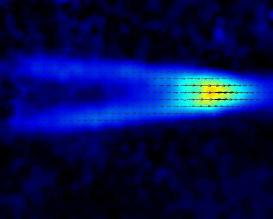# Vertical length scale selection for pancake vortices in strongly stratified viscous fluidsVertical length scale selection for pancake vortices in strongly stratified viscous fluids
R. Godoy-Diana; J. M. Chomaz & P. Billant.
Journal of Fluid Mechanics, 504 : 229-238 (2004).

Abstract: The evolution of pancake dipoles of different aspect ratio is studied in a stratified tank experiment. Two cases are reported here for values of the dipole initial aspect ratio $\alpha_0 = L_v/L_h$ (where $L_v$ and $L_h$ are vertical and horizontal length scales, respectively) of $\alpha_0 = 0.4$ (case I) and $\alpha_0 = 1.2$ (case II). In the first case, the usual decay scenario is observed where the dipole diffuses slowly with a growing thickness and a decaying circulation. In case II, we observed a regime where the thickness of the dipole decreases and the circulation in the horizontal mid-plane of the vortices remains constant. We show that this regime where the vertical length scale decreases can be explained by the shedding of two boundary layers at the top and bottom of the dipole that literally peel off vorticity layers. Horizontal advection and vertical diffusion cooperate in this regime and the decrease towards the viscous vertical length scale $\delta = L_h\hbox{\it Re}^{-1/2}$ occurs on a time scale $\alpha_0 \hbox{\it Re}^{1/2}T_A$, $T_A$ being the advection time $L_h/U$. From a scaling analysis of the equations for a stratified viscous fluid in the Boussinesq approximation, two dominant balances depending on the parameter $R = \hbox{\it Re}F_h^2$ are discussed, where $F_h = U/NL_h$ is the horizontal Froude number and $\hbox{\it Re} = UL_h/\nu$ is the Reynolds number, $U$, $N$ and $\nu$ being, respectively, the translation speed of the dipole, the Brunt–Väisälä frequency and the kinematic viscosity. When $R\gg 1$ the vertical length scale is determined by buoyancy effects to be of order $L_b = U/N$. The experiments presented in this paper pertain to the case of small $R$, where viscous effects govern the selection of the vertical length scale. We show that if initially $L_v \leq \delta$, the flow diffuses on the vertical (case I), while if $L_v \gg \delta$ (case II), vertically sheared horizontal advection decreases the vertical length scale down to $\delta$. This viscous regime may explain results from experiments and numerical simulations on the late evolution of stratified flows where the decay is observed to be independent of the buoyancy frequency $N$.

BibTeX:

@article{godoy-diana2004,
author = {{G}odoy-{D}iana, {R}. and {C}homaz, {J}. {M}. and {B}illant, {P}.},
title = {Vertical length scale selection for pancake vortices in strongly stratified viscous fluids},
journal = {Journal of Fluid Mechanics},
year = {2004},
volume = {504},
pages = {229-238}
}